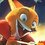Water Glass Challenge

This problem is inspired by the Problem of the Week.

A closed glass, shaped of a unit cube, consists of water $\frac{1}{6}$ filled. It is known that if we rotate the cube smoothly (so that the water does not "jump"), the water cross-section changes to one of some known shapes.

What is the volume of space, where the water cross-section will never reach throughout the rotation? For this problem, assume that the cross-section remains flat while changing its form.

Note: The answer is not $\frac{5}{6}$ since it is the volume of space where the water can't reach without rotating the cube.

Important: Please do not only post the answer. Share solutions!

If you are interested in using or borrowing this for your problem in the community, (as long as the correct answer doesn't appear yet) please let me know!Note by Michael Huang
1 year, 7 months ago

This discussion board is a place to discuss our Daily Challenges and the math and science related to those challenges. Explanations are more than just a solution — they should explain the steps and thinking strategies that you used to obtain the solution. Comments should further the discussion of math and science.

When posting on Brilliant:

• Use the emojis to react to an explanation, whether you're congratulating a job well done , or just really confused .
• Ask specific questions about the challenge or the steps in somebody's explanation. Well-posed questions can add a lot to the discussion, but posting "I don't understand!" doesn't help anyone.
• Try to contribute something new to the discussion, whether it is an extension, generalization or other idea related to the challenge.
• Stay on topic — we're all here to learn more about math and science, not to hear about your favorite get-rich-quick scheme or current world events.

MarkdownAppears as
*italics* or _italics_ italics
**bold** or __bold__ bold

- bulleted
- list

• bulleted
• list

1. numbered
2. list

1. numbered
2. list
Note: you must add a full line of space before and after lists for them to show up correctly
paragraph 1

paragraph 2

paragraph 1

paragraph 2

> This is a quote
This is a quote
# I indented these lines
# 4 spaces, and now they show
# up as a code block.

print "hello world"
# I indented these lines
# 4 spaces, and now they show
# up as a code block.

print "hello world"
MathAppears as
Remember to wrap math in $$...$$ or $...$ to ensure proper formatting.
2 \times 3 $2 \times 3$
2^{34} $2^{34}$
a_{i-1} $a_{i-1}$
\frac{2}{3} $\frac{2}{3}$
\sqrt{2} $\sqrt{2}$
\sum_{i=1}^3 $\sum_{i=1}^3$
\sin \theta $\sin \theta$
\boxed{123} $\boxed{123}$

Sort by:

If it is the volume of empty space that u r interested in knowing, it shall be nothing other than 5/6 cubic units. As the space taken by the 'emptiness' or water itself won't change at all, be it stationary or in motion

- 1 year, 1 month ago

I don't understand what you are asking.

- 1 year, 7 months ago

If the cube is fully rotated all the way around, you will notice the "empty" solid tangent to the water plane at some points. What is the volume of the space?

- 1 year, 7 months ago

Wouldn't that just be the remaining $\frac{5}{6}$?

- 1 year, 7 months ago

No, it is indeed not that much volume. I am referring to the central "holey" solid, where the cross section does not reach during the rotation. I am not referring to the empty prism for this problem.

I may consider revising the problem in case you interpret the problem incorrectly.

- 1 year, 7 months ago

Well, I would assume that the space would have 4/6 or 2/3 of a unit in diameter, so a 1/3 radius. When you do 4/3pi(r)^3 you get about 4/25. This is because the space within the cube is a sphere, correct?

- 1 year, 7 months ago

The solid within the cube is actually not the sphere since the slope of the cross-section changes relative to the total volume of the water. Taking the volume of space about the origin in the rectangular coordinate system, the radius is $\frac{1}{3}$ if the point touching it is $\frac{1}{6}$ units away from one of the cube's faces. It is intuitive that the radius of the space is $\frac{1}{3}$ as if the water cross-section is perpendicular throughout the rotation; however, this is not entirely true. Triangular pyramid of equilateral triangle is the popular example that disproves this.

Consider (1) the point closest to the centroid, and (2) the "sliced" cross-sections formed within the water. Two base cross-sections and a midsection should be helpful to answer the problem.

- 1 year, 7 months ago